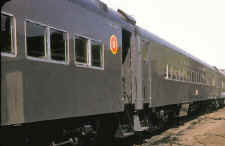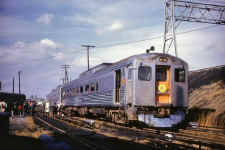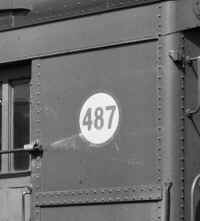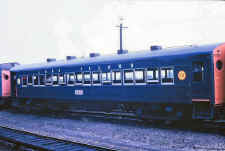LIRR Modernization Numbers LIRR MODERNIZATION NUMBERS (Compiled by Mike Boland, Art Single and Dave Keller) New Cars:  1-222 had red circle with yellow numbers and borders. Modernized Old Cars:  1-496 (+/-) had yellow circle with red numbers and borders. LIRR Modernization Numbers Roster LIRR's car modernization program and renumbering began in December, 1954.   Modernization numbers vs. Road numbers Primer Modernization numbers are the semi-random number assigned to the car AFTER rebuilding, and was stenciled in a circle near the vestibule doors and had NOTHING to do with the renumbering of the car's ROAD number.  For example: A MU car was #1153 and after modernization became #4153 road number, and was given a modernization number of #401 (in a round circle).  (See photo below in Part Two) Thus, the road number of #1153, makes it an MP54A1, according to Kohler's roster. Do not confuse road number with modernization number. Examples: If an MU car was numbered 401, for example, it became 4401 AFTER the renumbering. If an MU car was renumbered to 4153, for example, it was 153 PRIOR to renumbering. If a steam car was numbered 12, for example, it became 7012 AFTER the renumbering. If a steam car was renumbered to 7142, for example, it was 142 PRIOR to renumbering. As for abbreviations: MBM62 stood for Motor Baggage Mail (MU RPO and baggage car) and was 62 feet long. MPB54 stood for Motor Passenger Baggage (MU combine car) and was 54 feet long. MP54A, B, C, D were various MU motor passenger cars and were 54 feet long. Some had arched roofs, some had clerestory roofs. Research: Dave Keller Part One: NEW Cars (red circle with yellow numbers and borders)# 2906 modernization #5 P-72 Coach Photo: Anthony Debellis         Self-Propelled Rail Diesel Car:  Budd RDC 2 #3121 = 1Ā#3121 Morris Park 11/1960           Steam-type or Diesel-hauled cars: 2901-2925 = 2-26 2926-2980 = 136-190 MU Cars:  2501-2522 = 27-48 2601-2642 = 50-91 (note: 2642 was built a year after 2641) 2801-2844 = 92-135 2643-2674 = 191-222 Ā Self-Propelled Rail Diesel Car: Budd RDC 1 #3101 = 49LIRR #3101,  #3121 at Greenport 09/27/1959 Part Two: MODERNIZED Old Cars (yellow circle with red numbers and border)RPO Baggage Car #7737 close up of Modernization Number 487 Archive: Dave Keller Steam Cars P54D/E, PB57, P74:       6 = 242 1761 = 71 1856 = 66 7002 = 258 7014 = 75 7137 = 35    7393 = 27 7395 = 26 7397 = 23 7527 = 408 7534 = 415 7544 = 442 Steam Baggage & Bag/Mail Cars B60B, BM60, BM62: 724 = 126   (this car was later renumbered 7724 and wore the same mod. #) 7613 = 419 7617 = 384 7715 = 138 7716 = 315 7717 = 468 (note this differs from OBRM website, where they say 468 was 7738) 7719 = 169 7721 = 174 7724 = 126 7737 = 487 7738 = 394 (note this differs from OBRM website, where they say 7738 was 468) 7743 = 65 7753 = 432 7755 = 441 7756 = 446 MU Control Motor Cars MP54A/B/C/D, MP62, MBM62,MPB54 (renumbered into 4000 series): 4135 = 225 4146 = 226 4150 = 217 4153 = 401MP54D #401 7/22/2017 Photo: Michael Kam     4160 = 370 4171 = 349 4205 = 458 4206 = 481 4207 = 456 4209 = 477 4210 = 486 4212 = 125 4215 = 480 4381 = 461LIRR #4381 MPB-54 east end Babylon Yard 08-62 Photo: James Gillin           4692 = 79 4761 = 10LIRR #4761 MP54 Jamaica 08/1961 Photo: James Gillin             4835 = 187 4856 = 66   (circle number applied as car 1856 before modernizing) 4862 = 143 4900 = 30 4906 = 178 4938 = 41 4982 = 430 MU Motor Cars Lacking Controls and ASC MP54BT, MP54CT (renumbered into 5000 series): MU Trailer Cars T54A, T54B (renumbered into 6000 series): 6431 = 85 6855 = 496 Ā Rev. 07/12/2018R2-18 Westbound east of Lindenhurst, August 1964 Photo: Jim Gillin